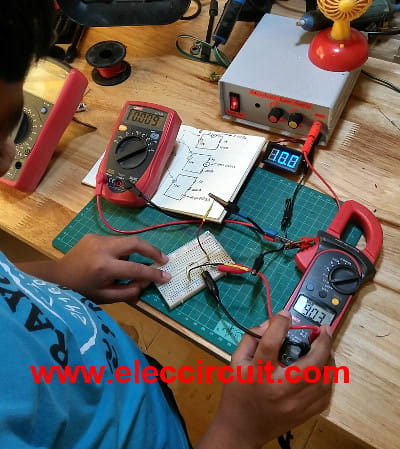# How to make a simple series circuit

When looks around the electronic circuit. What is the most type of circuits form? The series circuit is one of popular. Why? We are finding the answer. My son experiment on the simple series circuit. To understand current flow and voltage across all devices in the circuit.

Parts he will need
1. R2_1K—more than 0.25 watts resistors, tolerance: 5%
2. R1_100 ohms—Same with R2.
3. A variable DC power supply or 10V Fixed regulator.
4. A Breadboard—with at least 30 rows, inexpensive and useful.
6. Digital multimeter—voltmeter for finding the precise value of a voltage
7. Wire jumpers—use on a breadboard.

## Make simple series circuit

First of all, he builds the simple series circuit by using one resistor as Figure 1.
He installed all resistors into the breadboard. Then, apply the 10V power supply to the resistor-1K as a complete circuit.The simplest electronic circuit

In the circuit diagram, the voltage drop across R1-1K is 10V. Because it is connected in parallel with the battery, 10V.

But how many current R1 is using?

Then, he measures a current of the resistor-1K as Load of the circuit.
We will know current that flow in the resistor.Measure the current that flows through the resistor

Next, he connects the 100 ohms resistor in under 1K resistor as below.Measure voltage at the series form

He wants to know the voltage across each resistor.
So he uses the digital voltmeter measure voltage them.
The voltage across various resistor are different voltage, as follows.

• First, the voltage across R1-1K is 9.28V
• Second, the voltage across R2-2K is 0.933V
• And, the voltage across both resistors, R1+R2  are  9.28V and 0.933V = 10.17V
OR or this source voltage.

Then he measures current at various places.
As Figure 4The Current flows various resistors
R1 = 0.009A
R2 = 0.009A
R1+R2 = 0.009A
Thus, all parts have current equal 0.009A

As Figure 5. He study the series circuit by measuring voltage and current at various parts.Important features of the series circuit.
1. Electric current flowing through the same and have the same direction throughout the entire circuit.
2. The total resistance of the circuit is equal to the sum of the individual resistances in the circuit together.
3. The voltage across various parts of the circuit. When taken then combined will equal the voltage at the source.

This site uses Akismet to reduce spam. Learn how your comment data is processed.